# Week 2 - lecture 2

## TOP TOPIC

Date: 01/09/22

2.6. Mixing via coupling
2.7. The cycle and the Hypercube
2.8. Stationary Times
2.9. Strong Stationary Times and separation distance

## Homework

1.*  Letbe simple random walk on the hypercube, and let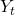denote the Hamming weight (i.e., the number of ones) of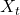.  Then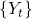is also a Markov chain, with transition probabilities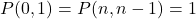and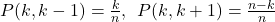for. Read about projected (or lumped) chains on pages 23-25 of the textbook, and calculatefor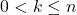. Hint: it suffices to calculate.

2.  Show that the mixing time of lazy simple random walk on the discrete torus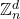(see section 5.3.3 and Theorem 5.6 of the book) is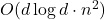.

3*.   Consider the following Markov chain on the integers:  Fix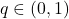. Letfor all integers. Let. For which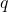is the hitting timefinite almost surely, and what is its expectation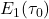?

A key issue is determining when the expectation is finite.  It is OK to use the strong law of large numbers.

4. Given an irreducible aperiodic transition matrix, show that for any two states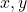, there is a coupling of the chains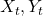started fromrespectively, such that the expected coalescence time of these chains is at most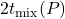.

5. Solve exercises 4.3 and 4.4 in the book, which can be found at https://www.yuval-peres-books.com/markov-chains-and-mixing-times/

## Reference

 Glasner, Shmuel.
Almost periodic sets and measures on the torus.
Israel J. Math. 32 (1979), no. 2-3, 161–-172.

 Berend, Daniel and Peres, Yuval.
Asymptotically dense dilations of sets on the circle.
J. London Math. Soc. (2) 47 (1993), no. 1, 1–-17.

 N. Alon and Y. Peres, "Uniform dilations'', Geometric and Functional Analysis, vol. 2, No. 1 (1992), 1–28.

 Nair, R. and Velani, S. L.
Glasner sets and polynomials in primes.
Proc. Amer. Math. Soc. 126 (1998), no. 10, 2835–-2840.